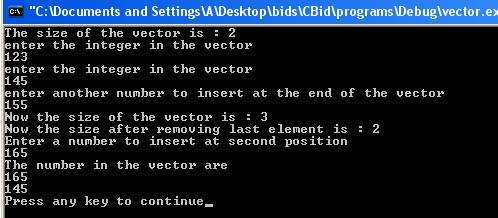Vector

A vector is a dynamic array. Vector is the most efficient way of implementing arrays. The functionality of vector is same as normal arrays used. The vectors automate the task of managing the size and capacity of the arrays. The new elements can be inserted easily. The elements can be erased with much less effort. In order to define vectors a header file vector is included in the program. The general form of a vector declaration is:-

vector<type>  variable_name;   or    vector<type> variable_name(size);

The type is the data type of the vector. The variable_name is the name of the variable. For example,

vector<int> a(10);

creates a vector a of integer type whose size is 10. Some of the functions of vector are:-

• Size- The function size() returns the size of the vector.
• Begin – The function begin() returns the iterator at the beginning of the vector.
• End – The function end()  returns the iterator at the end of the vector.
• Push Back- The function push_back() inserts an element at the end of the vector.
• Insert- The function insert() inserts an element in the middle of the vector.
• Erase- the function erase() remove the elements from the vector.
• Empty- The function empty() checks whether vector is empty or not. It returns true if vector is empty.
• Pop Back- The function pop_back() removes the last element from the vector.
• Resize – The function resize() resets the size of the vector.

Here is a program which illustrates the working of functions of vector.

#include<iostream>

#include<vector>

using namespace std;

int main ()

{

int i,num,num1=0 ;

vector<int> a(2);

vector<int>::iterator p;

if(a.empty())

{

cout << "Vector is now empty" << endl;

}

cout << "The size of the vector is : " << a.size() << endl;

for(i=0;i<2;i++)

{

cout << "enter the integer in the vector" << endl;

cin >> a[i];

}

cout << "enter another number to insert at the end of the vector" << endl;

cin >> num;

a.push_back(num);

cout << "Now the size of the vector is : " << a.size() << endl;

a.pop_back();

cout << "Now the size after removing last element is : "  << a.size() << endl;

cout << "Enter a number to insert at second position" << endl;

cin >> num1;

a.insert(a.begin()+1,num1);

p=a.begin();

a.erase(p,p+1);

cout << "The number in the vector are" << endl;

for(i=0;i<a.size();i++)

{

cout << a[i] << endl;

}

return(0);

}

The result of the program is:The statement

#include<vector>

includes a header file <vector> into the program. The statement

vector<int> a(2);

creates  a vector a of integer type of size 2. The statement

vector<int>::iterator p;

creates a iterator p for the vector.  The statement

if(a.empty)

checks whether the vector is empty. It returns false as the vector a is initialized with size 2. The statement

cout << "The size of the vector is : " << a.size() << endl;

displays the size of the vector. The function a.size() calculates the size of the vector which is currently 2.  The statement

a.push_back(num);

inserts the element num entered by the user at the end of the vector 3. The statement

cout << "Now the size of the vector is : " << a.size() << endl;

displays the current size of the vector which is 3 now after inserting element at the end of the vector. The statement

a.pop_back();

erases the last element from the vector a. The statement

cout << "Now the size after removing last element is : "  << a.size() << endl

displays the current size of the vector which is 2 after removing the last element from the vector. The statement

a.insert(a.begin()+1,num1);

inserts the element num1 entered at the second position of the vector. The function a.begin() places the iterator at the beginning of the vector. The function a.begin()+1 denotes the second position of the vector.  The statement

p=a.begin();

places the iterator p at the beginning of the vector. The statement

a.erase(p,p+1);

erases the first element from the vector. Now the vector size will be reduce by 1 and elements will shift to the one position before. In the end elements of the current vector are displayed.

Go to the previous lesson or proceed to the next lesson